# 别惹我，VLOOKUP是我哥

=LOOKUP(“々”,B:B)

=LOOKUP(“做”,B:B)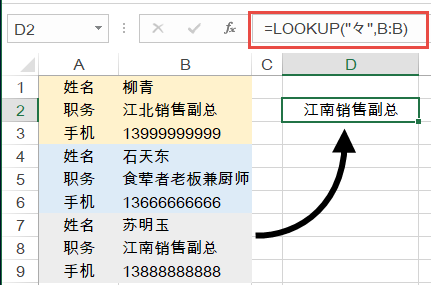=LOOKUP(9E+307,B:B)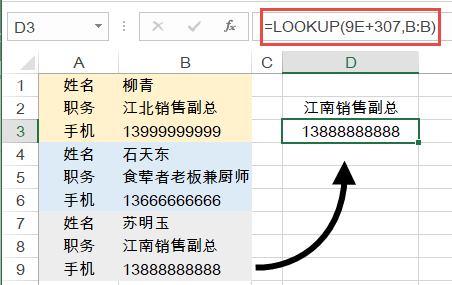=LOOKUP(“做”,B\$2:B2)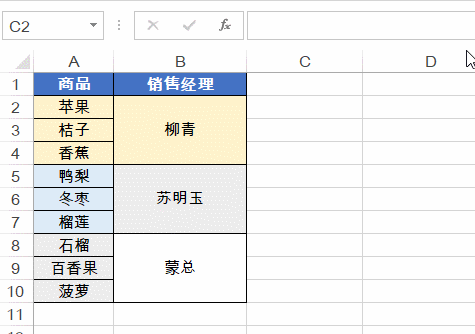=LOOKUP(1,0/(A:A<>””),A:A)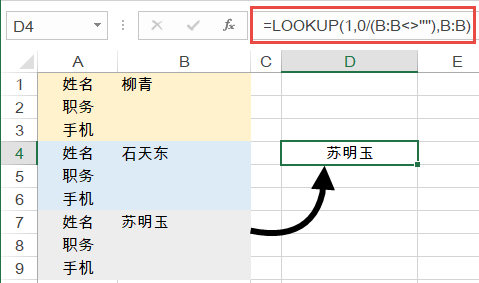=LOOKUP(1,0/(C2:C10=E3),A2:A10)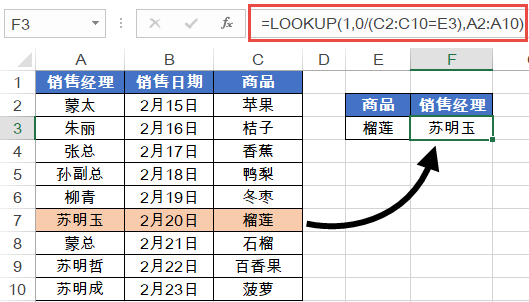=LOOKUP(1,0/(条件区域=条件),查询区域)

=LOOKUP(1,0/((D2:D10=F3)*(B2:B10=G3)),A2:A10)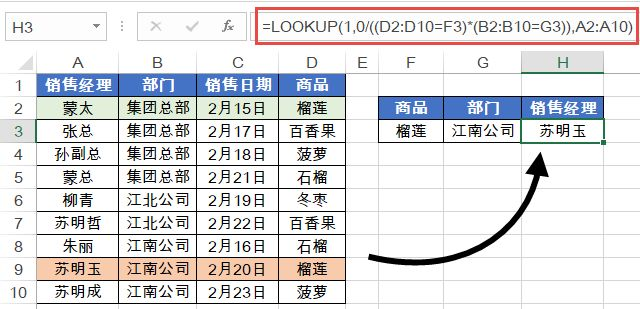=LOOKUP(1,0/((条件区域1=条件1)*(条件区域2=条件2)),查询区域)

C2单元格公式为：

=LOOKUP(B2,\$E\$3:\$F\$6)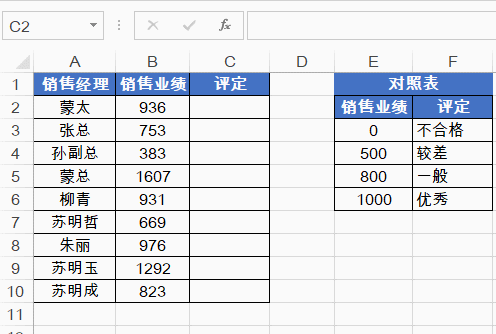=-LOOKUP(1,-RIGHT(B2,ROW(\$1:\$9)))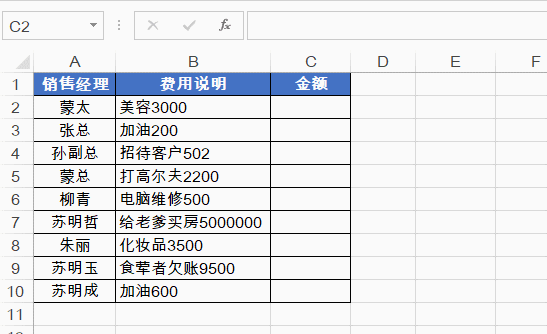LOOKUP函数使用1作为查询值，在由负数、0和错误值构成的数组中，忽略错误值提取最后一个等于或小于1的数值。最后再使用负号，将提取出的负数转为正数。

=LOOKUP(“做”,INDIRECT(“A1:A”&MATCH(D2,B1:B10,0)))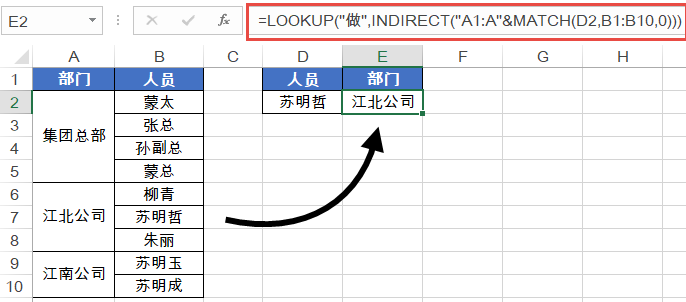MATCH(D2,B1:B10,0)部分，精确查找D2单元格的姓名在B列中的位置。返回结果为7。

=LOOKUP(“做”,A1:A7)